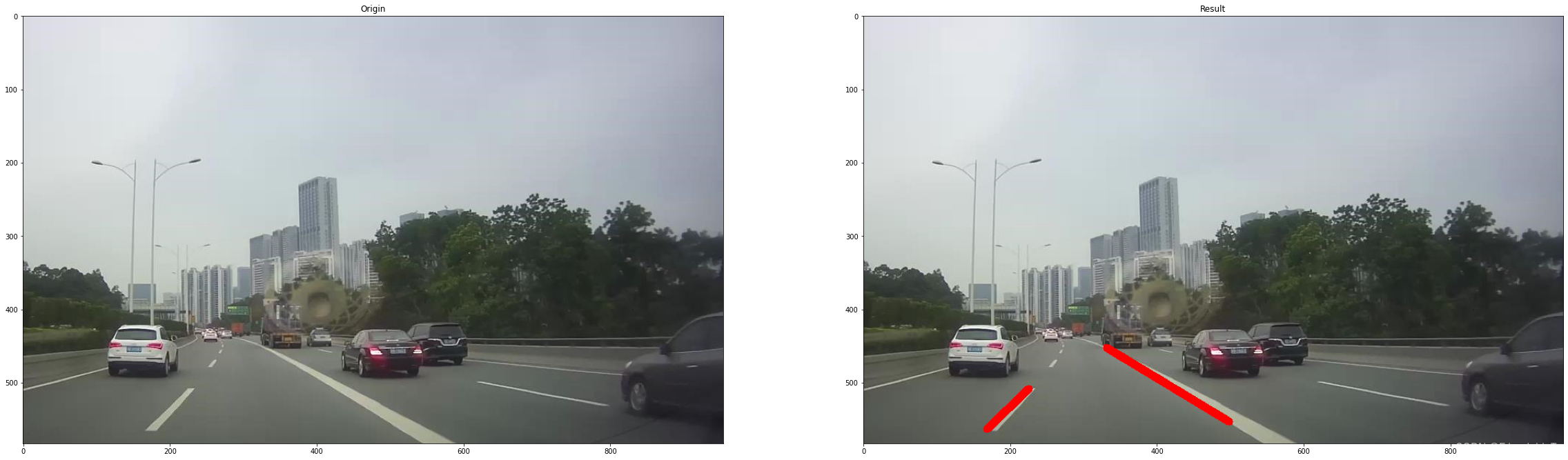# OpenCV-Python小应用（六）：车道线检测

### OpenCV-Python小应用（六）：车道线检测

• 前言
• 前提条件
• 实验环境
• 基于霍夫变换的车道线检测
• 参考文献

## 前言

• 本文是个人使用OpenCV-Python的应用案例，由于水平有限，难免出现错漏，敬请批评改正。
• 更多精彩内容，可点击进入OpenCV-Python小应用专栏或我的个人主页查看

• 熟悉Python

## 实验环境

• Python 3.6.13 （面向对象的高级语言）
• OpenCV 3.4.10（python第三方库）`pip3 install opencv-python==3.4.10.37`

## 基于霍夫变换的车道线检测

• 主要思路：利用霍夫变换将图片中的所有直线找出，并通过区域坐标过滤掉不是车道线的直线。这属于传统算法的范畴，有一定的局限性。
• 霍夫变换相关知识点，可查阅OpenCV-Python快速入门（十五）：霍夫变换
``````import cv2
import numpy as np
import matplotlib.pyplot as plt

# 读取图片
# 灰度图
gray = cv2.cvtColor(img,cv2.COLOR_BGR2GRAY)
# 边缘检测
edges = cv2.Canny(gray,50,150,apertureSize = 3)
# BGR -> RGB
img_RGB=cv2.cvtColor(img,cv2.COLOR_BGR2RGB)
img_Show=img_RGB.copy()
# 概率霍夫变换
'''
lines =cv2.HoughLinesP(image, rho, theta, threshold, minLineLength, maxLineGap)

image 是输入图像，即源图像，必须为 8 位的单通道二值图像。
对于其他类型的图像，在进行霍夫变换之前，需要将其修改为这个指定的格式。
rho 为以像素为单位的距离 r 的精度。一般情况下，使用的精度是 1。
theta 是角度𝜃的精度。一般情况下，使用的精度是 np.pi/180，表示要搜索可能的角度。
threshold 是阈值。该值越小，判定出的直线越多；值越大，判定出的直线就越少。
minLineLength 用来控制“接受直线的最小长度”的值，默认值为 0。
maxLineGap 用来控制接受共线线段之间的最小间隔，即在一条线中两点的最大间隔。
如果两点间的间隔超过了参数 maxLineGap 的值，就认为这两点不在一条线上。默认值为 0。

lines 是由 numpy.ndarray 类型的元素构成的，其中每个元素都是一对浮点数，表示检测到的直线的参数，即(r, θ)。
'''
lines = cv2.HoughLinesP(edges,1,np.pi/180,1,minLineLength=55,maxLineGap=9)

for line in lines:
x1,y1,x2,y2 = line
if x1 < 150 or x1 > 500 or x2 > 500 or y2 < 500: # 过滤多余的直线
continue
# print(x1,y1,x2,y2)
cv2.line(img_RGB,(x1,y1),(x2,y2),(255,0,0),10)

# 显示图片
plt.figure(figsize=(40, 40))
plt.subplot(121)
plt.title("Origin")
plt.imshow(img_Show)
plt.subplot(122)
plt.title("Result")
plt.imshow(img_RGB)
plt.show()
``````## 参考文献

 https://opencv.org/
 李立宗. OpenCV轻松入门：面向Python. 北京： 电子工业出版社，2019

• 更多精彩内容，可点击进入OpenCV-Python小应用专栏或我的个人主页查看